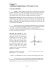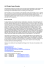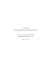# Chapter 1. Newton`s Laws of Motion

## Transcription

Chapter 1. Newton`s Laws of Motion
```Chapter 1.
Newton’s Laws of Motion
Notes:
• Most of the material in this chapter is taken from Young and Freedman, Chapters
4 and 5
1.1 Forces and Interactions
It was Isaac Newton who first introduced the concepts of mass and force, to a large
extent to make sense of the experimental results obtained by previous scientists. Using
these concepts, or principles, he was able to put forth three fundamental laws of motions
(i.e., Newton’s Laws of Motion) upon which much of classical physics rests upon1. We
review the different types of forces encountered in Newtonian (or classical) mechanics
before we introduce Newton’s Laws.
A force is an interaction between two bodies or between a body and its environment. One
intuitive type of force is a contact force, which often clearly involves a direct interaction
(or contact) between the surfaces or boundaries of the bodies involved. We can further
discriminate between different kinds of contact forces.
1. A body in contact with the surface of another object will experience a force that
is directed normal to that surface. Perhaps the simplest example of a normal
force is that of a book resting on a table. Since the book does not fall under its
own weight it must be that the table is exerting a force normal to its surface to
keep the book at rest. Another example is that of a block at rest or sliding on an
inclined plane. Even in the case where the block is sliding, there is the presence
of a force oriented perpendicularly to the surface of the plane; if not the block
would not be sliding but falling through the plane.
2. The aforementioned normal force is not the only one exerted by the plane on an
object sliding on it. There is a friction force that is oriented parallel to the
surface of contact, but in the direction opposite to that of the sliding. Friction
forces can be further differentiated depending on whether the object is not sliding
(but is about to) or it is already in motion. More precisely, it takes a greater
applied force to start the object moving (i.e., to overcome the static friction
force) than it takes to keep it sliding (when the kinetic friction force is at work).
3. Finally, whenever an object is pulled, through a string or a rope attached to it, it
can be set in motion through a tension force (as long as it is stronger than the
static friction force).
1
Exceptions to this include anything involving elementary particles or atoms and
molecules (i.e., the realm of quantum mechanics) as well as classical electromagnetism.
The inconsistency of Newton’s Laws with Maxwell’s equations of electromagnetism led
to the development of special relativity, by A. Einstein, which expands upon (and
corrects) Newton’s Laws to situations involving motions nearing the speed of light.
-1-
Contact forces are not the only agents through which bodies interact, however. For
example, electric and magnetic forces as well as the gravitational force act over distances
in vacuum and therefore do not require any type of contact (or a series of contacts) to
make themselves felt by bodies. Such forces are referred to as long-range forces.
1.1.1 The Principle of Superposition
Evidently more than one force or type of force can applied at once to an object. One can
imagine that our block sliding on an inclined plane through the influence of gravity could
at the same time be slowed downed through the use of a rope pulled by someone standing
above the block on the plane. Such a block would then be subjected to four different
forces: a normal force, a friction force, a tension force, and a long-range (gravitation)
force. The question arises then as to how these forces combine when acting on a body. As
it turns out their combined effect is additive2. That is, we can apply the principle of
superposition and add all the forces vectorially (since forces are vectors, i.e., they have a
magnitude and a direction).
Mathematically this is expressed as follows, given a set of n forces Fi for i = 1, 2, …, n
acting on an object the resultant or net force R felt by this object is
n
R = ∑ Fi .
(1.1)
i=1
When expressed using Cartesian coordinates the forces can be broken with their
components along the x-, y-, and z-axis such that
n
Rx = ∑ Fx,i
i=1
n
Ry = ∑ Fy,i
(1.2)
i=1
n
Rz = ∑ Fz,i .
i=1
For example, for the case of our previous sliding block we have
R = N + f + T + G,
(1.3)
where N, f, T, and G are the normal, friction, tension, and gravitational forces,
respectively.
2
Here we assume that the body can be appropriately modeled as a single point or that all
the forces are applied at its centre of mass.
-2-
1.2 Newton’s Laws
Now that we have determined the kind of forces that are susceptible to affect the
dynamics of bodies in Newtonian mechanics, we now turn to the laws of nature that will
allow us to quantify these interactions. Newton’s Laws are often simply stated as:
I. A body remains at rest or in uniform motion unless acted upon by a net force.
II. A body acted upon by a net force moves in such a manner that the time rate of
change of the momentum equals the force.
III. If two bodies exert forces on each other, these forces are equal in magnitude and
opposite in direction.
The First Law would be meaningless without the concept of force, but it conveys a
precise meaning for the concept of a “zero net force”. This tendency for a body to remain
in its initial state of motion (or at rest) is called inertia. One should note that according to
the first law, there is no way to distinguish between “no net force” and “no force at all”.
That is, the only thing that matter is the resultant. It is irrelevant whether two or three
forces (or any number for that matter) are applied simultaneously, if they cancel each
other out, then their effect (or lack thereof) is the same as that of having no force at all
applied to the body; the body will remain in its initial state of uniform motion. We then
say that the body is in equilibrium (since it is not influenced by forces).
The Second Law is very explicit: Force is the time rate of change of the momentum. But
what is the momentum p …
p ≡ mv,
(1.4)
with m the mass, and v the velocity of the body. We therefore rewrite the Second Law as
dp
dt
d
= ( mv ) .
dt
Fnet =
(1.5)
Although we still don't have a definition for the concept of mass, we can further
transform equation (1.5), if we assume that it is a constant, to yield
dv
dt
= ma,
Fnet = m
(1.6)
with a ≡ dv dt the acceleration resulting from the action of the net force on the body.
Note that the acceleration is in the same direction as the force and proportional to it (the
constant of proportionality being the mass).
-3-
The concept of mass is made clear with the Third Law, which can be rewritten as
follows:
III'. If two bodies constitute an ideal, isolated system, then the accelerations of these
bodies are always in opposite direction, and the ratio of the magnitudes of the
accelerations is constant. This constant ratio is the inverse ratio of the masses of
the bodies.
If we have two isolated bodies, 1 and 2, then the Third Law states that
F1 = −F2 ,
(1.7)
dp1
dp
=− 2,
dt
dt
(1.8)
m1a1 = −m2 a 2
m1 a2
= ,
m2 a1
(1.9)
and from the Second Law we have
or using the acceleration a
with ai = a i . If one chooses m1 as the reference or unit mass, m2 , or the mass of any
other object, can be measured by comparison (if it is allowed to interact with m1 ) of their
measured accelerations. Incidentally, we can use equation (1.8) to provide a different
interpretation of Newton’s Second Law
d
( p1 + p 2 ) = 0
dt
(1.10)
p1 + p 2 = constant.
(1.11)
or
The momentum is conserved in the interaction of two isolated particles. This is a special
case of the conservation of linear momentum, which is a concept that we will discuss at
length later on.
One should note that the Third Law is not a general law of nature. It applies when dealing
with central forces (e.g., gravitation (in the non-relativistic limit), electrostatic, etc.), but
not necessarily to other types of forces (e.g., velocity-dependent forces such as between
to moving electric charges). But such considerations are outside the scope of our studies.
-4-
1.2.1 Inertial Frames of Reference
The concept of an inertial frame of reference is central to the application of Newton’s
Laws. An inertial frame is one that is in a uniform and non-accelerating state of motion.
In fact, Newton’s Laws are only applicable to such frame of references. Conversely,
Newton’s First Law can be used to define what an inertial frame is.
For example, suppose that a body that is not subjected to any net force is in a dynamical
state that is in accordance with the First Law, as seen by an observer at rest in a given
frame of reference. One would therefore define this frame as being inertial (for that
reason Newton’s First Law is often call the law of inertia). Now if a second observer at
rest in another frame sees that the body is not moving in a uniform motion, then this
second frame of reference cannot be inertial. This may due, for example, to the fact that
this frame of reference is itself accelerating in some fashion, which would account for the
apparent non-uniform motion of the observed body. It is often stated that Newton’s Laws
are invariant when moving from one inertial frame to another. This can be better
understood mathematically.
If a particle of mass m has a velocity u′ relative to an observer who is at rest an inertial
frame K ′ , while this frame is moving with at a constant velocity v as seen by another
observer at rest in another inertial frame K (note the necessity of having a constant
relative velocity v ), then we would expect that the velocity u of the particle as measured
in K to be
u = u′ + v.
(1.12)
That is, it would seem reasonable to expect that velocities should be added together when
transforming from one inertial frame to another3 (such a transformation is called a
Galilean transformation). If we write the mathematical form of the Second Law in
frame K we have
du
dt
d ( u′ + v )
=m
,
dt
(1.13)
du′
= F′.
dt
(1.14)
F=m
but since v is constant
F=m
3
The development of special relativity has showed that this law for the composition of
velocities is approximate and only valid when these are small compared to the speed of
light.
-5-
The result expressed through equation (1.14) is a statement of the invariance (or
covariance) of Newton’s Second Law. More precisely, the Second Law retains the same
mathematical form no matter which inertial frame is used to express it, as long as
velocities transform according to the simple addition rule stated in equation (1.12).
1.2.2 Exercises
1. (Prob. 4.8 in Young and Freedman.) You walk into an elevator, step onto a scale, and
push the “up” button. You also recall that your normal weight is 625 N. Start answering
each the following questions by drawing a free-body diagram.
a) If the elevator has an acceleration of magnitude of 2.50 m/s 2 , what does the scale
b) If you start holding a 3.85-kg package by a light vertical string, what will be the
tension in the string once the elevator begins accelerating?
Solution.
4-4
4.8.
a) The elevator and everything in it are accelerating upward, so we apply Newton’s
Second Law in the vertical direction only. Your mass is determined with
m = w g = 625 N 9.8 N kg −1 = 63.8 kg , but you and the package have the same
acceleration as the elevator. Taking +y as the upward direction we use the freebody diagram of Figure 1a), where n is the scale reading, we calculate
Chapter 4
∑
IDENTIFY: The elevator and everything in it are F
accelerating
upward, so we apply Newton’s second law
y = n−w
(1.15)
in the vertical direction.
SET UP: Your mass is m = w/g = 63.8 kg. Both you=and
mathe package have the same acceleration as the
elevator. Take + y to be upward, in the direction of the acceleration of the elevator, and apply
Fy =or
ma y .
EXECUTE: (a) Your free-body diagram is shown in Figure 4.8a, where n is the scale reading.
n = w + ma
Fy = ma y
gives n − w = ma. Solving for n gives n = w + ma = 625 N + (63.8 kg)(2.50 m/s 2 ) = 784 N.
= 625isNgiven
+ 63.8
kg ⋅ 2.50
(b) The free-body diagram for the package
in Figure
4.8b.
2
784
T = w + ma = (3.85 kg)(9.80 m/s 2 + =
2.50
m/sN.
) = 47.4 N.
2
m/s
F = ma
y
y
gives T − w = ma, so
Figure 4.8
Figure 1 – Free-body diagram.
4.9.
EVALUATE: The objects accelerate upward so for each of them the upward force is greater than the
downward force.
IDENTIFY: Apply F = ma to the box.
SET UP: Let + x be the direction of the force and acceleration. Fx = 48.0 N.
48.0 N
Σ Fx
=
= 16.0 kg.
ax
3.00 m/s-2 6 EVALUATE: The vertical forces sum to zero and there is no motion in that direction.
IDENTIFY: Use the information about the motion to find the acceleration and then use
EXECUTE:
4.10.
calculate m.
Fx = ma x gives m =
Fx = ma x to
(1.16)
b) Referring to the free-body diagram of Figure 1b) we write
∑F
y
= T − wp
= mp a
(1.17)
or
T = wp + mp a
= 3.85 kg ⋅ ( 9.80 m/s 2 + 2.50 m/s 2 )
(1.18)
= 47.4 N.
2. (Prob. 4.57 in Young and Freedman.) Two boxes, A and B, are connected to each end
of a light rope (see Figure 2). A constant upward force of 80.0 N is applied to box A.
Starting from rest, box B descends 12.0 m in 4.00 s. The tension in the rope connecting
the two boxes is 36.0 N. What are the masses of the two boxes?
Solution.
The system is accelerating, so we apply Newton’s second law to each box and can use the
constant acceleration kinematics for formulas to find the acceleration. We now that
d 2x
a= 2
dt
(1.19)
t
τ
x ( t ) = ∫ ⎡⎢ ∫ a ( λ ) d λ ⎤⎥ dτ
−∞ ⎣ −∞
⎦
(1.20)
and therefore
Figure 2
In one dimension (along the y-axis ) when the acceleration is constant equation (1.20)
becomes
-7-
1
y = y0 + v0 yt + ayt 2 ,
2
(1.21)
where y0 and v0 y are, respectively, the position and velocity at t = 0 . We therefore have
for box B ( v0 y = 0 )
2 ( y − y0 )
t2
2 ⋅ ( −12.0 m )
=
16 s2
= −1.5 m/s2 .
ay =
(1.22)
Alternatively we can write (defining g > 0 )
mB ay = T − mB g,
(1.23)
or
mB =
=
T
g + ay
36.0 N
( 9.8 − 1.5 ) m/s2
(1.24)
= 4.34 kg.
And for box A
mA ay = F − T − mA g,
(1.25)
or
mA =
=
F −T
g + ay
( 80.0 − 36.0 ) N
( 9.8 − 1.5 ) m/s2
(1.26)
= 5.30 kg.
Note that even though the boxes have the same acceleration they experience different
forces because they have different masses.
-8-
(b) Σ Fy = 0 gives n cos11.0° − w = 0 and n =
w
. Σ Fx = 0 gives F − n sin11.0° = 0 and
cos11.0°
Figure
w 5.9a, b
F=
sin11.0° = w tan11.0° = 343 N.
.0° : Apply Newton’s first law to the hanging weight and to each knot. The tension force at each
5.10.
Icos11
DENTIFY
end of a string is the same.
(a) Let the tensions in the three strings be T, T ′, and T ′′, as shown in Figure 5.10a.
Figure 5.10a
SET UP: The free-body diagram for the block is given in Figure 5.10b.
EXECUTE:
Figure 3 – System for Problem 3 (left) and free-body
Σ Fy = 0
diagram (right).
T′ − w = 0
w = 60.0 N .
3. (Prob. 5.10 in Young and Freedman.) In Figure 3 the weight
T ′ = w = 60.0 N
5.9a, b in the diagonal string?
a) What isFigure
the tension
5.10. the
IDENTIFY
: Applyof
Newton’s
first law to the
hanging
to each knot. The tension force at each
b) Find
magnitudes
the horizontal
forces
F1 weight
and Fand
2 that must be applied to
end ofFigure
a string5.10b
is the same.
hold the(a)system
the position
shown.
Let the in
tensions
in the three
strings be T, T ′, and T ′′, as shown in Figure 5.10a.
Solution.
No portion of this material may be reproduced, in any form or by any means, without permission in writing from the publisher.
Newton’s first law will suffice for this problem as the system is in equilibrium. We can
apply it to the hanging weight and to each knot. The tension force at each end of a string
is the same.
a) Let the tensions in the three strings be T , T ′, and T ′′ , as shown in Figure 3. The
free-body
diagram
Figure
5.10a for the block is
SET UP: The free-body diagram for the block is given in Figure 5.10b.
EXECUTE:
Σ Fy = 0
T′ − w = 0
T ′ = w = 60.0 N
Figure 5.10b
We can therefore write
No portion of this material may be reproduced, in any form or by any means, without permission in writing from the publisher.
∑F
y
= T′−w
= 0,
or T ′ = w = 60.0 N . The free-body diagram for the lower knot is
-9-
(1.27)
Applying Newton’s Laws
5-5
SET UP: The free-body diagram for the lower knot is given in Figure 5.10c.
EXECUTE:
ΣFy = 0
T sin 45° − T ′ = 0
T′
60.0 N
T=
=
= 84.9 N
sin 45° sin 45°
And we have
Figure 5.10c
(b) Apply Σ Fx = 0 to the force diagram for the lower knot:
ΣFx = 0
∑F
y
= T sin ( 45 ) − T ′
Applying Newton’s Laws
F2U=PT: cos
45free-body
° = (84.9 N)cos
= 60
N
SET
The
diagram
the.0lower
knot is given in Figure 5.10c.
= 0,45°for
SET UP: The free-body diagram for the upper knot is E
given
in Figure
5.10d.
XECUTE
:
5-5
(1.28)
ΣE
FXECUTE
=0 :
Applying Newton’s Laws
y
which yields T = 2T ′ = 84.9 N .
Fx45
=°0− T ′ = 0
TΣ
sin
b) We now apply Newton’s first law in the x direction. ForT the
lower
knot
we have
cosT45
F60
′in° −
N
SET UP: The free-body diagram for the lower knot is given
Figure
1 =.005.10c.
T
=
=
=
84.9 N
from the above figure
45.°9 N)cos
sin 4545
°°
F sin
= (84
∑F
1 EXECUTE:
ΣF60
F1 =
0 0N
y .=
T sin 45° − T ′ = 0
(1.29)
Figure 5.10c
T′
60.0 N
= 0,
T=
=
= 84.9 N
Figure 5.10d
(b) Apply Σ Fx = 0 to the force diagram for the lower knot:
sin 45° sin 45°
Note that F1 = F2 .
ΣF2x =
= 060.0 N . For the upper knot we have the following free-body
EVALUATE: Applying Σ Fy = 0 to the upper knot gives T ′′ = T sin 45° = 60.0 N = w. If we treat the whole
F2 = T cos 45° = (84.9 N)cos 45° = 60.0 N
system
a5.10c
single
object,
the force
given
Figure
5.10e. 5.10d.
Figure
SET
UP: asThe
free-body
diagram
for diagram
the upperisknot
is in
given
in Figure
x
or F2 = T
diagram
= T cos ( 45 ) − F2
5-5
gives F:2 = F1, which checks
(b) Apply Σ Fx = 0 to the force diagram for theΣFlower
x =0
Eknot:
XECUTE
ΣFy = 0ΣFgives
x = 0 T ′′ = w, which checks
ΣFx = 0
F2 = T cos 45° = (84.9 N)cos 45° = 60.0 N
T cos 45° − F1 = 0
SET UP: The free-body diagram for the upper knot is
Figure45
5.10d.
F1 given
= (84.in
9 N)cos
°
XECUTE
F1 =E60
.0 N :
ΣFx = 0
Figure 5.10e
5.11.
T cos
° − F1 = 0in the rocket. A constant force
IDENTIFY: We apply Newton’s second law to the rocket and
the45
astronaut
F1 = (84.9 N)cos 45°
Note
= F2free-body
.
SETthat
UP: F1The
diagrams for the rocket (weight wrF) and
astronaut (weight w) are given in
1 = 60.0 N

′′
EVALUATE
: Applying
Σ
F
=
0
to
the
upper
knot
gives
T
=
T
sin
45
°the
= 60
.0 N =
w. If on
wethe
treat
the whole
y
Figures 5.11a
and 5.11b.
F
is
the
thrust
and
n
is
the
normal
force
rocket
exerts
astronaut.
The
T ′′ = T sin 45
Figure
5.10d
With a similar
calculation
we find that F1 =soFwe
60.0
N . Finally, we also see that
2 =can
means we have constant acceleration,
use the standard kinematics equations.
T
( )
(1.30)
system
singleisobject,
theWe
force
in Figure 5.10e.
speed as
of asound
331 m/s.
usediagram
ΣFy = is
magiven
y and v = v0 + at .
= 60.0 N.
Figure 5.10d
ΣFx = 0 gives F2 = F1, which checks
Note that F1 = F2 .
ΣF = 0 gives T ′′ = w, which checks
y
These last twoEVALUATE
results :could
have been easily predicted
by treating the whole
Applying Σ Fy = 0 to the upper knot gives T ′′ = T sin 45° = 60.0 N = w. If we treat the whole
system as a single object and studying the corresponding force diagram
system as a single object, the force diagram is given in Figure 5.10e.
ΣFx = 0 gives F2 = F1, which checks
Figure 5.10e
5.11.
ΣFy = 0 gives T ′′ = w, which checks
IDENTIFY: We apply Newton’s second law to the rocket and the astronaut in the rocket. A constant force
means we have constant acceleration, so we can use the standard kinematics equations.
SET
UP: 5.11
The free-body diagrams for the rocket (weight wr ) and astronaut (weight w) are given in
Figure
Figures
5.11a
and 5.11b. FT is the thrust and n is the normal force the rocket exerts on the astronaut. The
Figure
5.10e
speed
of
sound
331apply
m/s. We
use ΣFsecond
v =rocket
v0 + atand
. the astronaut in the rocket. A constant force
y = malaw
y and
5.11.
IDENTIFY: isWe
Newton’s
to the
means
wemay
havebeconstant
acceleration,
use without
the standard
kinematics
No portion of this
material
reproduced,
in any form orsobywe
anycan
means,
permission
in writingequations.
from the publisher.
SET UP: The free-body diagrams for the rocket (weight wr ) and astronaut (weight w) are given in
Figures 5.11a and 5.11b. FT is the thrust and n is the normal force the rocket exerts on the astronaut. The
- 10
speed of sound is 331 m/s.
We -use ΣFy = ma y and v = v0 + at .
1.3 Friction Forces Revisited
We have already briefly discussed friction forces. We now seek to quantify them in
relation to another contact force, i.e., the normal force. The nature of the friction force is
very complicated. At its basis, it is a microscopic phenomenon involving the making and
breaking of molecular bonds between the two contacting surfaces. It should therefore not
be expected that any friction force is constant over time as an object is sliding on a
surface (obviously we are referring here to the kinetic friction force). This is because the
number of bonds created or destroyed will vary depending on the roughness of the
surfaces at different position or the inhomogeneous presence of alien matter between
them (e.g., dirt or oil). It follows that our assigning of a single, constant force for a given
problem is an approximation, i.e., it must represent some sort of macroscopic average
that results from the detailed microphysical phenomena that take place.
1.3.1 Kinetic Friction
It is found experimentally that the kinetic friction force is proportional to the normal
force of contact. If fk and n represent these two forces, then they can be related through
fk = µ k n ,
(1.31)
where µ k is the coefficient of kinetic friction. The norms (  ) in equation (1.31) are
not used and this relation will commonly be written as
fk = µ k n.
(1.32)
It should be noted that neither of equations (1.31) or (1.32) are vectorial in nature, since
fk and n are perpendicular to one another.
1.3.2 Static Friction
As was stated earlier, friction does not imply motion. In fact, it is usually the case that
friction is stronger when an objet is immobile. More precisely, the static friction force fs
on a body is normally stronger than the kinetic friction that settles in once it starts
moving. We know from experiments that the maximum magnitude that the static friction
force can take, ( fs )max , is proportional to the normal contact force. It follows that
fs ≤ µ s n,
(1.33)
where µ s is the coefficient of static friction. Experimental measurements show that
typical values range (approximately) from 0.05 < µ s < 1 (but it can be greater than unity),
while µ k < µ s in general.
- 11 -
1.3.3 Rolling Friction
Interestingly, although the less friction there is between a body on a surface the better the
former slides on the latter, the same is not true for rolling motions. That is, a good rolling
action depends on the presence of some friction; rolling would not take place in the limit
where there is no friction. On the other hand, rolling friction and its corresponding
coefficient or rolling friction µr , defined as the ratio of the force needed for constant
speed to the normal force exerted by the surface over which the rolling motion takes
place, is significantly lower than the coefficient of kinetic friction µ k . Typical values for
µr range from 10 −3 to 10 −2 . If you have to move a heavy object, you may do well to put
wheels under it …
1.3.4 Fluid Resistance and Friction
The definition of a fluid can encompass a whole variety of agent. A fluid could be loosely
defined as a substance (gas or liquid) that deforms under shear stress and easily yields to
external pressure. Some examples include the atmosphere (air), oils, or the rarefied
agglomerations of matter (i.e., gases made of molecules and dust) in the interstellar
medium.
If we take the case of an object in motion in the atmosphere, one could model this (solid)
body-fluid interaction by considering the many collisions involving the molecules that
make the atmosphere on the surface of the body. These interactions will transfer linear
momentum between the colliding partners and affect the motion and kinetics of the body
as it progresses on its path through the fluid. We have yet to study collisions in detail but
the motion of an object within a fluid can nonetheless be determined experimentally.
For objects moving a low speed relative to the surrounding fluid the friction, or drag
force resulting from the numerous body-fluid collisions is found to be proportional to the
speed v
f = kv,
(1.34)
with k the proportionality constant. This friction force is directed in opposition to the
direction of motion. For larger objects moving at higher speeds (on the order of, say, 10
m/s) the drag force is proportional to the square of the velocity
f = Dv 2 .
(1.35)
Newton’s Second Law can be used to gain more insight, so let us consider an object of
mass m sinking into a fluid under the effect of gravity. We therefore find
mg − f = ma.
- 12 -
(1.36)
For motions occurring at low speed where equation (1.34) applies the solutions to
equation (1.36) for the velocity v ( = dy dt ) and the position y as a function of time can
mg
1− e−kt m )
(
k
mg ⎡ m
⎤
y (t ) =
t − (1− e−kt m ) ⎥ ,
⎢
k ⎣
k
⎦
v (t ) =
(1.37)
respectively, for t > 0 . The acceleration is given by (also when t > 0 )
dv
dt
= ge−kt m .
a=
(1.38)
We find that the velocity, which we assume to be v = 0 at t = 0 , eventually reaches a
maximum, terminal velocity when t = ∞ (when a = 0 , obviously)
vt =
mg
.
k
(1.39)
Since this terminal velocity is constant it could have easily been determined with
equation (1.36) when equilibrium is reached (i.e., when a = 0 ). We would then have that
mg − kvt = 0,
(1.40)
from which we recover equation (1.39). The non-linear nature of the friction force at
higher speed (i.e., equation (1.35)) renders it impossible to calculate solutions similar to
those presented in equations (1.37). But we can still consider equilibrium conditions to
determine a terminal velocity
vt =
mg
.
D
(1.41)
The existence of a terminal velocity is entirely due to the fact that the friction force scales
with the velocity of the object. This is to be contrasted with the case of contact friction
forces, which are independent of the velocity.
1.4 Circular Motions
Consider an object that is initially moving with a uniform, rectilinear motion at velocity
v . One might ask what force must be applied to the object such that it enters into a
circular orbit of radius R at the same speed. Referring to Figure 4 we see that over an
- 13 -
dθ
v dt
dr
R
R
dθ
Figure 4 – Motion of a body over an infinitesimal amount of
time . To go from a rectilinear to a circular motion of radius
, its linear path must be modified by an amount
.
infinitesimal time dt the object must “fall” a (radial) distance dr towards the centre of
the circular orbit.
Again referring to the figure, we can write
dr = ( v dt ) dθ
(1.42)
v dt = R dθ .
(1.43)
and
We will now define two quantities: the angular velocity
dθ
dt
v
=
R
ω≡
(1.44)
and the radial acceleration that the body must have in order to get into the circular orbit
dt
1 ⎛ dr ⎞
= ⎜ ⎟
dt ⎝ dt ⎠
=v
dθ
dt
which when using equation (1.44) becomes
- 14 -
(1.45)
v2
R
= ω 2 R.
(1.46)
This radial acceleration is more commonly known as the centripetal acceleration.
Because an object moving in uniform motion will remain as such unless subjected to a
non-zero net force (Newton’s First Law), circular motion (with a constant orbital
velocity) will only be realized if a net force oriented radially toward the centre of the
orbit act on the object to induce a centripetal acceleration as defined in equation (1.46).
This net force must enter Newton’s Second Law when dealing with problems involving
circular motions.
1.4.1 Exercises
4. (Prob. 5.42 in Young and Freedman) A small car with a mass of 0.8 kg travels at
constant speed on the inside of a track that is a vertical circle with a radius of 5.0 m. If the
normal force exerted by the track on the car when it is at the top of the track is 6.0 N,
what is the normal force on the car when it is at the bottom of the track? What is the
speed of the car?
Solution.
Two forces are acting on the car, gravity and the normal force. At the top, both forces are
toward the center of the circle, so Newton’s second law gives
mg + nB = marad .
(1.47)
At the bottom, gravity is downward but the normal force is upward, so
nA − mg = marad .
(1.48)
Solving equation (1.47) for the acceleration
nB
m
= 9.8 m/s +
6N
0.8 kg
= 17.3 m/s 2 .
We can now determine that
- 15 -
(1.49)
nA = m ( g + arad )
= 0.8 kg ( 9.8 + 17.3) m/s 2
(1.50)
= 21.7 N.
The normal force at the bottom is greater than at the top because it must balance the
weight in addition to accelerate the car toward the center of its track. Finally, the speed of
the car is found to be (with equation (1.46)
= 17.3 m/s 2 ⋅ 5 m
= 9.3 m/s.
- 16 -
(1.51)
```

### PHY131H1F - Class 9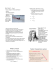### Symmetry-breaking due to Coulomb friction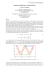### Tribotester - ASEC Kunststoffen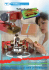### Rock Shoes Tech Sheet 2011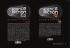### Saturday, October 29, 2016 9 am - 4 pm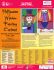### Equipments and Materials for Tribotesting in Open Space on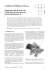### Cybex Trazer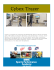### THANDIE NEWTON CHIWETEL EJIOFOR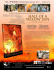### SMART FUN in MIRABILANDIA ( )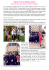### Introductory module: a video introduction to Modellus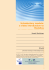### Lecture PowerPoints Chapter 5 Physics: Principles with Applications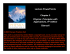### The Crossings Connection October 2011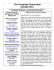### NewtoN`s Laws of MotioN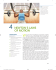### braintwister v2.0